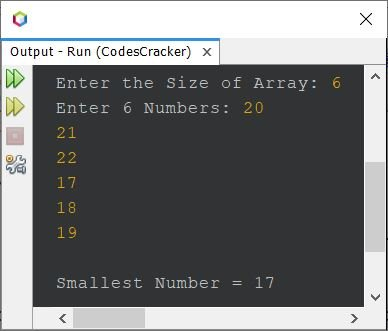# Java Program to Find Smallest Number in an Array

This article is created to cover a program in Java that find and prints the smallest number in an array of n numbers or elements, entered by user at run-time.

## Java Find Smallest Number in Array using for Loop

The question is, write a Java program to find the smallest number in an array of n numbers. The program given below is its answer:

```import java.util.Scanner;

public class CodesCracker
{
public static void main(String[] args)
{
int tot, i, small;
Scanner scan = new Scanner(System.in);

System.out.print("Enter the Size of Array: ");
tot = scan.nextInt();
int[] arr = new int[tot];
System.out.print("Enter " +tot+ " Numbers: ");
for(i=0; i<tot; i++)
arr[i] = scan.nextInt();

small = arr;
for(i=1; i<tot; i++)
{
if(small>arr[i])
small = arr[i];
}

System.out.println("\nSmallest Number = " +small);
}
}```

The snapshot given below shows the sample run of above program with user input 6 as size and 20, 21, 22, 17, 18, 19 as six numbers, to find and print the smallest number among these:## Java Find Smallest Number in Array using while Loop

To create the same program using while loop, instead of for. Then replace the following block of code, from above program:

```for(i=1; i<tot; i++)
{
if(small>arr[i])
small = arr[i];
}```

with the block of code given below:

```i = 0;
while(i<tot)
{
if(small>arr[i])
small = arr[i];
i++;
}```

#### Same Program in Other Languages

Java Online Test

« Previous Program Next Program »